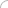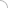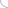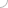# Volume and surface area - aptitude test

Home > Aptitude test > Volume and surface area

##### 1) What is the mass of the man, if a boat of length 4m, width 1.5m floating on a river sinks by 0.015 m when the man gets on the boat?

a) 100kg
b) 90kg
c) 80kg
d) 70kg

SOLUTION :

Volume of water displaced = 4*1.5*0.015 = 0.09m3
Mass = volume of water displaced * density of water
Mass = 0.09 * 1000
Mass = 90kg

##### 2) For a rectangular box, what is the product of the areas of the 3 adjacent faces that meet at a point?

a) Volume of the box
b) Twice the volume of the box
c) Half of the volume of the box
d) Square of the volume of the box

SOLUTION :

The area of the 3 adjacent faces of a rectangular box of length ‘l’, breadth ‘b’, and depth ‘h’ are ‘l*b’, ‘b*h’, and ‘l*h’.
Therefore, lb*bh*lh = l2b2h2 = (lbh) 2 = square of the volume of the box.

##### 3) Find the number of cubes of 2cm edge will be required to fill a cubical box of 2m edge.

a) 105
b) 103
c) 104
d) 106

SOLUTION :

Volume of the box = N*volume of the cubes … (N = number of cubes)
23 = N*(0.02) 3
8 = N*(0.000008)
N = 106

##### 4) What is the ratio of the volumes of a right cylinder to that of a right cone having equal diameters?

a) 1:3
b) 3:1
c) 2:3
d) 3:2Aptitude questions